###### How to Solve Word Problems Programmatically

Chapter

3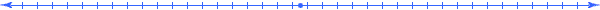Chapter 3.  Abstraction

 3.1 Real Things 3.2 Abstract Things 3.3 Dimensions of Real Things 3.4 Dimensions of Abstract Things 3.5 Constructing Shapes 3.6 Relating Shapes 3.7 Relating Angles 3.8 Activities and Explorations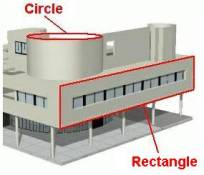3.1

#### Real Things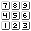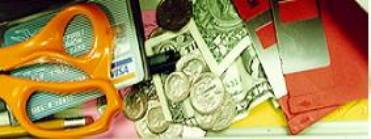Real Things

The concept of a "thing" comes from that which we experience in our lives.  Things include not only physical objects, but other concepts as well such as events in time, monetary transactions, software programs, etc.  In this book, we will focus on things that we can measure.

3.2

#### Abstract Things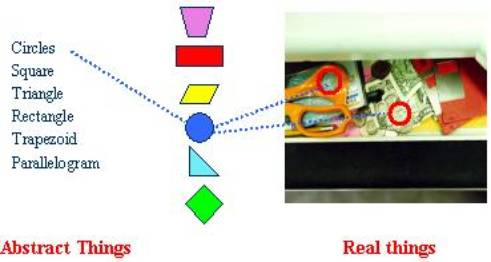Abstract Things Describe Real Things

Abstract things help us to describe and relate real things.  The abstract concept of a circle can describe the shape of a coin or the hole in scissor handles,

 When we associate an abstract thing like a "sphere" to a real thing like a "baseball", then the dimensions (like radius, diameter, surface area, volume, etc.) of that abstract thing apply to the real thing "baseball".  We can develop general relationships between the dimensions of abstract things and apply them to all real things associated with them.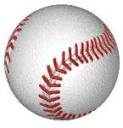3.3

#### Dimensions of Real Things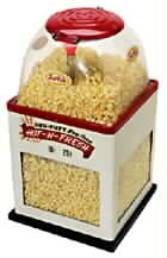[cm~Height(Popper)] [in~Width(Popper)] [ft~Length(Popper)] [dol~ManufacturingCost(Popper)] [yen~WholesaleCost(Popper)] [euro~RetailPrice(Popper)] [oz~Weight(Popper)] [ins~Occurrence(PoppersSold)] [ins~Occurrence(PoppersOrdered)] [ins~Occurrence(Corn)] [L~Volume(Popper)] [ºC~Temperature(Popper)] Scaled Dimensions of A Real Thing

Things usually have many properties, and the properties that we can measure (with a unit scale) we call dimensions.  This includes not only geometric dimensions such as width, height, and depth; but also such dimensions as weight, temperature, cost, surface area, volume, speed, time, density, surface area, etc.  Note how the width, height, and depth dimensions of an appliance (like a washer machine) correspond to an abstract rectangular solid (a box) with minimal size to contain the appliance.

3.4

#### Dimensions of Abstract Things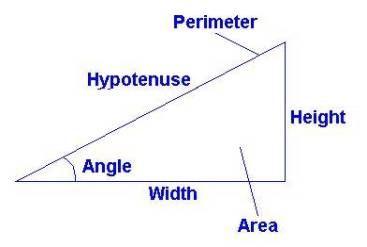Dimensions of a Right Triangle [m~Height(Triangle)] [m~Width(Triangle)] [m~Hypotenuse(Triangle)] [m~Perimeter(Triangle)] [m~Area(Triangle)] [m~Angle(Triangle)] Scaled Dimensions Associated with a Triangle

A triangle is an example of an abstract thing.  It has many related dimensions.  The dimensions listed here appear a basic algebra course.  But with a little bit of curiosity, browse through many other properties of triangles at http://mathworld.wolfram.com/Triangle.html.  It may surprise you how many dimensions you will find.  Similarly for dimensions of circles, check out http://mathworld.wolfram.com/Circle.html

3.5

#### Constructing ShapesPoint Line Ray   Circle     Right Angle   Parallel Lines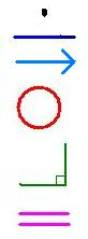The Fundamental Building Blocks of Classical Geometry

In the 4-th century BC, a brilliant teacher Euclid set up a system of geometric objects in his famous work, The Elements.  In essence, he began with some basic shapes and construction concepts (points, lines, rays, circles, right angles, parallel lines) and then constructed and proved the field of classical geometry.  Used for over two millenniums as the primary resource for math education, The Elements presents logical arguments to construct many of the abstract things and relationships found in mathematics.  (http://aleph0.clarku.edu/~djoyce/java/elements/toc.html)

 The proof of Proposition 1 illustrates Euclid's construction approach.  From his five fundamental postulates, one can construct a line between two points and a circle around a point.  From these he shows how to construct an equilateral triangle using a line between two points that are the centers of two circles with that line as their radius.  See more details at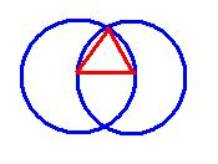Proposition 1. Construction of Equilateral Triangle

It is amazing how Euclid began with 23 definitions and 5 postulates in Book I and then proceeded to prove over 465 propositions beginning with such simple concepts of point, line, circle, and angle.   Indeed, geometry gives us many abstract shapes and relationships between those shapes that help us describe the world around us.  These basic shapes allow us to create many interesting geometric figures and properties.  Explore the interactive examples of constructions at the website:  http://www15.addr.com/~dscher/

Even today, geometry textbooks teach the concept of "proof", and build propositions and theorems based on assumed definitions and postulates.

3.6

#### Relating Shapes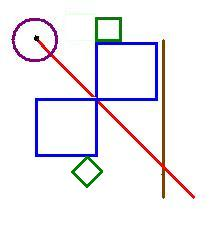Center Above Adjacent Intersect Parallel Similar Congruent Reflection Rotation Symmetric Related Geometric Figures

Certain words in the mathematical vocabulary describe shapes (figures) and the relationships between those geometric shapes.  In the above diagram, use the listed words to describe patterns that you see.  For resources to do this visit:  http://www.mathleague.com/help/geometry/coordinates.htm

3.7

#### Relating Angles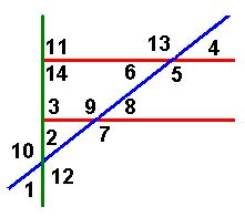Right Complimentary Supplementary Adjacent Acute Obtuse Vertical Alternate interior Alternate exterior Corresponding Angle Vocabulary Words

Certain words in the mathematical vocabulary describe types of angles and the relationships between angles.  The above diagram contains two horizontal parallel lines with two other lines, one perpendicular and one oblique (having a slant).  Use the listed words to describe relationships and patterns in this diagram.  For resources to do this visit:  http://www.mathleague.com/help/geometry/angles.htm

3.8

#### Activities and ExplorationsActivities:

 Play “Reality” game Either draw or find examples of a real things associated with each word in a given mathematical vocabulary list.  Such a list can come from the other team or you may want to start with a list of vocabulary words needed for Math Competency and SAT exams in Basic Algebra Vocabulary Words.   Play “Abstract Scavenger Hunt” game Have each team search a particular area and record as many abstract things and dimensions that they can apply to real objects they find.  The team with the most things and dimensions in their list wins.   Play “Clueless” game - Be the last person to state a new clue (a unified quantity) for a known object within a specific time.  (No dimension can be repeated).

Explorations:

 Review Basic Geometry Terms and Concepts   Construct Geometric Shapes from Other Shapes   Explore Euclid's Elements http://aleph0.clarku.edu/~djoyce/java/elements/toc.html

Exercises:

 Do Related Problems In Your Textbook   Using Meaning Table Use a Meaning Table to solve word problems in

Copyright © 2004  Dr. Ranel E. Erickson